## Sunday, October 29, 2017

### New fix to optimization in `ratebytree` for comparing diversification rates

I just updated (1, 2) the phytools function `ratebytree` for comparing the rates of speciation & extinction between trees.

I noticed that (as I described earlier today for `fit.bd`) occasionally during optimization of the different diversification models, `ratebytree` would return non-finite values of the log-likelihood.

Since this almost always seems to occur far from the genuine ML values, the easiest thing to do was to merely add a check to verify that the log-likelihood was finite, and, if not, repeat optimization with different starting values for the parameters of the model until the optimization succeeds or until a certain number of iterations has been reached.

It seems to work. Here I'll demonstrate with the old function version - then I'll load from source & show that it works. Obviously, the user should just update phytools from GitHub rather than loading the function from source!

``````library(phytools)
library(TreeSim)
``````

First, a set of trees that fails under `model="equal-extinction"`:

``````set.seed(304)
b<-(log(50)-log(2))/100
trees<-sim.bd.taxa.age(n=50,numbsim=3,lambda=b,mu=0,frac=1,age=100,T)
class(trees)<-"multiPhylo"
ratebytree(trees,model="equal-extinction")
``````
``````## Warning in nlminb(c(init.b, 0), lik.eqmu, t = t, rho = rho, trace =
## trace, : NA/NaN function evaluation
``````
``````## ML common diversification-rate model:
##  b   d   k   log(L)
## value    0.0385  0.0108  2   -200.9624
##
## ML multi diversification-rate model:
##  b    b    b    d    d    d    k   log(L)
## value    0.1527  0.3209  0.7647  0.6047  0.6047  0.6047  4   Inf
##
## Diversification model was "equal-extinction".
## Model fitting method was "nlminb".
##
## Likelihood ratio: Inf
## P-value (based on X^2): 0
``````
``````## load from source
source("https://raw.githubusercontent.com/liamrevell/phytools/master/R/ratebytree.R")
ratebytree(trees,model="equal-extinction")
``````
``````## Warning in nlminb(c(init.b, 0), lik.eqmu, t = t, rho = rho, trace =
## trace, : NA/NaN function evaluation
``````
``````## Warning in nlminb(c(init.b, init.d), lik.onerate, t = t, rho = rho, model =
## model, : NA/NaN function evaluation
``````
``````## ML common diversification-rate model:
##  b   d   k   log(L)
## value    0.0385  0.0108  2   -200.9624
##
## ML multi diversification-rate model:
##  b    b    b    d    d    d    k   log(L)
## value    0.0379  0.0383  0.0392  0.0107  0.0107  0.0107  4   -200.9466
##
## Diversification model was "equal-extinction".
## Model fitting method was "nlminb".
##
## Likelihood ratio: 0.0317
## P-value (based on X^2): 0.9843
``````

Now a set that fails under `model="equal-speciation"`:

``````rm(list=ls())
set.seed(9)
b<-(log(50)-log(2))/100
trees<-sim.bd.taxa.age(n=50,numbsim=3,lambda=b,mu=0,frac=1,age=100,T)
class(trees)<-"multiPhylo"
ratebytree(trees,model="equal-speciation")
``````
``````## Warning in nlminb(c(init.b, rep(0, length(trees))), lik.eqlam, t = t, rho =
## rho, : NA/NaN function evaluation
``````
``````## Warning in nlminb(c(init.b, init.d), lik.onerate, t = t, rho = rho, model =
## model, : NA/NaN function evaluation
``````
``````## ML common diversification-rate model:
##  b   d   k   log(L)
## value    0.0332  0.0017  2   -204.3688
##
## ML multi diversification-rate model:
##  b    b    b    d    d    d    k   log(L)
## value    0.3515  0.3515  0.3515  0.9476  0   0   4   Inf
##
## Diversification model was "equal-speciation".
## Model fitting method was "nlminb".
##
## Likelihood ratio: Inf
## P-value (based on X^2): 0
``````
``````## load from source
source("https://raw.githubusercontent.com/liamrevell/phytools/master/R/ratebytree.R")
ratebytree(trees,model="equal-speciation")
``````
``````## Warning in nlminb(c(init.b, rep(0, length(trees))), lik.eqlam, t = t, rho =
## rho, : NA/NaN function evaluation
``````
``````## Warning in nlminb(runif(n = 2, 0, 2) * c(init.b, rep(0, length(trees))), :
## NA/NaN function evaluation
``````
``````## Warning in nlminb(c(init.b, init.d), lik.onerate, t = t, rho = rho, model =
## model, : NA/NaN function evaluation
``````
``````## ML common diversification-rate model:
##  b   d   k   log(L)
## value    0.0332  0.0017  2   -204.3688
##
## ML multi diversification-rate model:
##  b    b    b    d    d    d    k   log(L)
## value    0.0334  0.0334  0.0334  0.0058  0   0   4   -204.1787
##
## Diversification model was "equal-speciation".
## Model fitting method was "nlminb".
##
## Likelihood ratio: 0.3803
## P-value (based on X^2): 0.8268
``````

Since fixing `fit.bd` I am not able to find failing cases for `model="birth-death"`, and I also could not find failing cases for the common-rates model, but I added the fix anyway.

That's it.

### Fix to optimization routine for non-finite log-likelihoods in `fit.bd`

I recently added the function `fit.bd` to theh phytools package. This function fits a birth-death model & essentially duplicates existing function in ape and diversitree.

I discovered, however, that sometimes optimization fails even for this simple model, and it seems to be usually due to numerical issues which causes the log-likelihood to evaluate to a non-finite value during optimization. This can result in some weird discrepancies between optimized birth (λ) and death (μ) rates between phytools and ape.

This is what I mean:

``````library(phytools)
library(TreeSim)
set.seed(1)
trees<-sim.bd.taxa.age(n=100,numbsim=200,lambda=0.059,
mu=0.020,frac=1,age=100,mrca=TRUE)
fits<-lapply(trees,fit.bd)
``````
``````## There were 9 warnings (use warnings() to see them)
``````
``````## birth & death rates from ape
BD.ape<-t(sapply(trees,function(x) bd(birthdeath(x))))
## birth & death rates from phytools
BD.phytools<-t(sapply(fits,function(x)
setNames(c(x\$b,x\$d),c("b","d"))))
plot(BD.ape[,"b"],BD.phytools[,"b"],cex=1.5,pch=21,bg="grey",
xlab=expression(paste("ape::birthdeath  ",hat(lambda))),
ylab=expression(paste("phytools::fit.bd  ",hat(lambda))))
text(BD.ape[,"b"],BD.phytools[,"b"],1:length(fits),cex=0.5,pos=1)
title(main=expression(paste("Estimated speciation rates, ",lambda,
", from ape & phytools")))
``````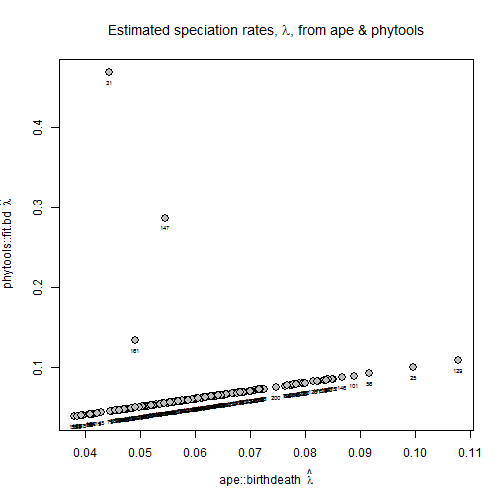``````plot(BD.ape[,"d"],BD.phytools[,"d"],cex=1.5,pch=21,bg="grey",
xlab=expression(paste("ape::birthdeath  ",hat(mu))),
ylab=expression(paste("phytools::fit.bd  ",hat(mu))))
text(BD.ape[,"d"],BD.phytools[,"d"],1:length(fits),cex=0.5,pos=1)
title(main=expression(paste("Estimated extinction rates, ",mu,
", from ape & phytools")))
``````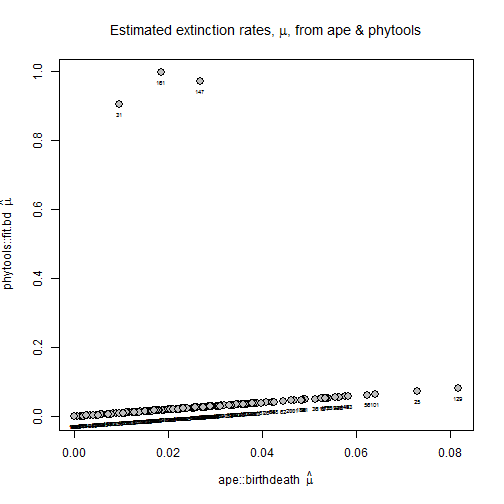We can immediately see that three trees, #31, #147,& #161, have estimated speciation & extinction rates that are way off!

If we look at their log-likelihoods it turns out they are all non-finite:

``````sapply(fits[c(31,147,161)],logLik)
``````
``````##  Inf Inf Inf
``````

The simple fix that I have pushed so far is to simply check if the optimized log-likelihood is finite, and then, if not, to re-run the optimization routine with different starting values (or until a certain number of iterations is reached).

Let's load it & try it. (Note that the user should just update phytools from GitHub).

``````source("https://raw.githubusercontent.com/liamrevell/phytools/master/R/bd.R")
qb<-phytools:::qb ## make internal function visible
fits<-lapply(trees,fit.bd)
``````
``````## There were 32 warnings (use warnings() to see them)
``````
``````b<-sapply(fits,function(x) x\$b)
par(mar=c(5.1,5.1,4.1,2.1))
plot(BD.ape[,"b"],b,cex=1.5,pch=21,bg="grey",
xlab=expression(paste("ape::birthdeath  ",hat(lambda))),
ylab=expression(paste("phytools::fit.bd  ",hat(lambda))))
title(main=expression(paste("Estimated speciation rates, ",lambda,
", from ape & (fixed) phytools")))
``````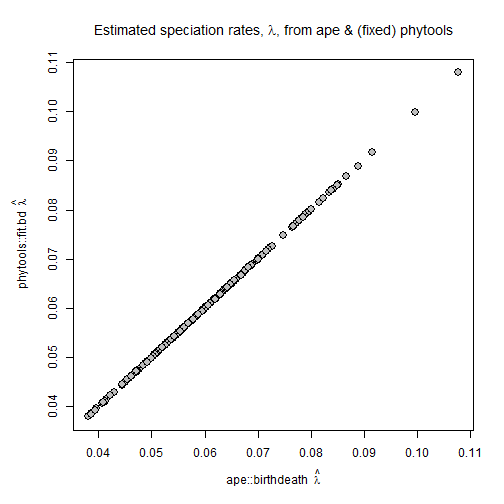Good!

### Two more models for comparing the diversification rates between reconstructed phylogenies

I just pushed (also here) a significant addition to the phytools function `ratebytree` for `type="diversification"` which allows the user to compare diversification rates (speciation & extinction) between phylogenies (e.g., 1, 2).

This update now permits us to fit two additional models: one (`model="equal-extinction"`) in which speciation is permitted to vary among trees, but not extinction; and a second (`model="equal-speciation"`) in which extinction may vary, but not speciation.

In the following, I'll demonstrate this with two sets of simulated trees as follows:

``````library(phytools)
packageVersion("phytools") ## from GitHub
``````
``````##  '0.6.36'
``````
``````print(tt1,details=TRUE)
``````
``````## 3 phylogenetic trees
## tree 1 : 500 tips
## tree 2 : 500 tips
## tree 3 : 500 tips
``````
``````print(tt2,details=TRUE)
``````
``````## 3 phylogenetic trees
## tree 1 : 500 tips
## tree 2 : 500 tips
## tree 3 : 500 tips
``````

First, let's visualize the branching times in our first set of trees, `tt1`:

``````obj<-ltt(tt1,plot=FALSE)
xlim<-c(max(sapply(obj,function(x) max(x\$times))),0)
ylim<-c(1,max(sapply(obj,function(x) max(x\$ltt))))
plot(max(obj[]\$times)-obj[]\$times,obj[]\$ltt,xlim=xlim,
ylim=ylim,log="y",type="s",xlab="time before present",
ylab="lineages",col="red",lwd=2,lend=1)
lines(max(obj[]\$times)-obj[]\$times,obj[]\$ltt,
col="orange",lwd=2,lend=1,type="s")
lines(max(obj[]\$times)-obj[]\$times,obj[]\$ltt,
col="blue",lwd=2,lend=1,type="s")
text(max(obj[]\$times),1.1,"tree 1",pos=4)
text(max(obj[]\$times),1.1,"tree 2",pos=4)
text(max(obj[]\$times),1.1,"tree 3",pos=4)
title(main="Equal speciation / variable extinction")
``````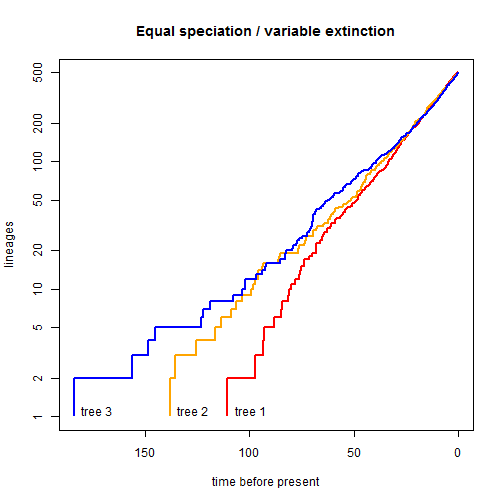Now let's fit our different models. In total, we have four: a model in which speciation & extinction rates are equal between trees, a second in which speciation rates vary but extinction rates are equal between phylogenies, a third in which the converse is true, and, finally, a fourth in which birth & death rates are both allowed to vary among phylogenies.

``````eqex<-ratebytree(tt1,model="equal-extinction")
``````
``````## Warning in nlminb(c(init.b, 0), lik.eqmu, t = t, rho = rho, trace =
## trace, : NA/NaN function evaluation
``````
``````## Warning in nlminb(c(init.b, init.d), lik.onerate, t = t, rho = rho, model =
## model, : NA/NaN function evaluation
``````
``````eqex
``````
``````## ML common diversification-rate model:
##          b       d       k   log(L)
## value    0.0491  0.0104  2   1652.323
##
## ML multi diversification-rate model:
##          b    b    b    d    d    d    k   log(L)
## value    0.0524  0.0486  0.0465  0.0101  0.0101  0.0101  4   1654.3604
##
## Diversification model was "equal-extinction".
## Model fitting method was "nlminb".
##
## Likelihood ratio: 4.0748
## P-value (based on X^2): 0.1304
``````
``````eqsp<-ratebytree(tt1,model="equal-speciation")
``````
``````## Warning in nlminb(c(init.b, init.d), lik.onerate, t = t, rho = rho, model =
## model, : NA/NaN function evaluation
``````
``````eqsp
``````
``````## ML common diversification-rate model:
##          b       d       k   log(L)
## value    0.0491  0.0104  2   1652.323
##
## ML multi diversification-rate model:
##          b    b    b    d    d    d    k   log(L)
## value    0.0486  0.0486  0.0486  7e-04   0.0097  0.0163  4   1657.5991
##
## Diversification model was "equal-speciation".
## Model fitting method was "nlminb".
##
## Likelihood ratio: 10.5522
## P-value (based on X^2): 0.0051
``````
``````bd<-ratebytree(tt1)
``````
``````## Warning in nlminb(c(init.b, init.d), lik.bd, t = T, rho = rho, lower =
## rep(0, : NA/NaN function evaluation

## Warning in nlminb(c(init.b, init.d), lik.bd, t = T, rho = rho, lower =
## rep(0, : NA/NaN function evaluation
``````
``````bd
``````
``````## ML common diversification-rate model:
##          b       d       k   log(L)
## value    0.0491  0.0104  2   1652.323
##
## ML multi diversification-rate model:
##          b    b    b    d    d    d    k   log(L)
## value    0.0471  0.0475  0.0546  0   0.0081  0.0243  6   1658.7793
##
## Diversification model was "birth-death".
## Model fitting method was "nlminb".
##
## Likelihood ratio: 12.9127
## P-value (based on X^2): 0.0117
``````
``````AIC(eqex,eqsp,bd)
``````
``````##                                   AIC df
## common-rate                 -3300.646  2
## multi-rate:equal-extinction -3300.721  4
## multi-rate:equal-speciation -3307.198  4
## multi-rate:birth-death      -3305.559  6
``````
``````aic.w(setNames(AIC(eqex,eqsp,bd)[,1],
c("common-rate","equal-extinction","equal-speciation",
"birth-death")))
``````
``````##      common-rate equal-extinction equal-speciation      birth-death
##       0.02489281       0.02584185       0.65896525       0.29030009
``````

This shows us that (by a small margin) the favored model is one in which all three trees share a common speciation rate - but differ in their fitted extinction rates.

Now let's try again with our second set of phylogenies. First plotting them:

``````print(tt2,details=TRUE)
``````
``````## 3 phylogenetic trees
## tree 1 : 500 tips
## tree 2 : 500 tips
## tree 3 : 500 tips
``````
``````obj<-ltt(tt2,plot=FALSE)
xlim<-c(max(sapply(obj,function(x) max(x\$times))),0)
ylim<-c(1,max(sapply(obj,function(x) max(x\$ltt))))
plot(max(obj[]\$times)-obj[]\$times,obj[]\$ltt,xlim=xlim,
ylim=ylim,log="y",type="s",xlab="time before present",
ylab="lineages",col="red",lwd=2,lend=1)
lines(max(obj[]\$times)-obj[]\$times,obj[]\$ltt,
col="orange",lwd=2,lend=1,type="s")
lines(max(obj[]\$times)-obj[]\$times,obj[]\$ltt,
col="blue",lwd=2,lend=1,type="s")
text(max(obj[]\$times),1.1,"tree 1",pos=4)
text(max(obj[]\$times),1.1,"tree 2",pos=4)
text(max(obj[]\$times),1.1,"tree 3",pos=4)
title(main="Equal extinction / variable speciation")
``````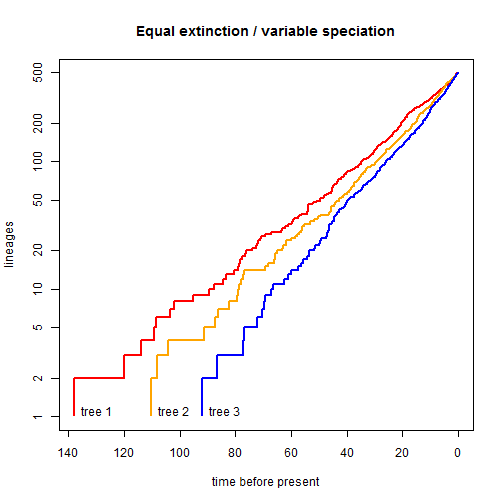Now once again we can fit our four different models & compare them:

``````eqex<-ratebytree(tt2,model="equal-extinction")
``````
``````## Warning in nlminb(c(init.b, init.d), lik.onerate, t = t, rho = rho, model =
## model, : NA/NaN function evaluation
``````
``````eqex
``````
``````## ML common diversification-rate model:
##          b       d       k   log(L)
## value    0.0612  0.0148  2   1951.3546
##
## ML multi diversification-rate model:
##          b    b    b    d    d    d    k   log(L)
## value    0.0514  0.0599  0.0701  0.0115  0.0115  0.0115  4   1964.3627
##
## Diversification model was "equal-extinction".
## Model fitting method was "nlminb".
##
## Likelihood ratio: 26.0162
## P-value (based on X^2): 0
``````
``````eqsp<-ratebytree(tt2,model="equal-speciation")
``````
``````## Warning in nlminb(c(init.b, init.d), lik.onerate, t = t, rho = rho, model =
## model, : NA/NaN function evaluation
``````
``````eqsp
``````
``````## ML common diversification-rate model:
##          b       d       k   log(L)
## value    0.0612  0.0148  2   1951.3546
##
## ML multi diversification-rate model:
##          b    b    b    d    d    d    k   log(L)
## value    0.0604  0.0604  0.0604  0.0229  0.012   0   4   1958.951
##
## Diversification model was "equal-speciation".
## Model fitting method was "nlminb".
##
## Likelihood ratio: 15.1928
## P-value (based on X^2): 5e-04
``````
``````bd<-ratebytree(tt2)
``````
``````## Warning in nlminb(c(init.b, init.d), lik.onerate, t = t, rho = rho, model =
## model, : NA/NaN function evaluation
``````
``````bd
``````
``````## ML common diversification-rate model:
##          b       d       k   log(L)
## value    0.0612  0.0148  2   1951.3546
##
## ML multi diversification-rate model:
##          b    b    b    d    d    d    k   log(L)
## value    0.0489  0.0595  0.0756  0.0068  0.0106  0.0216  6   1965.0754
##
## Diversification model was "birth-death".
## Model fitting method was "nlminb".
##
## Likelihood ratio: 27.4415
## P-value (based on X^2): 0
``````
``````AIC(eqex,eqsp,bd)
``````
``````##                                   AIC df
## common-rate                 -3898.709  2
## multi-rate:equal-extinction -3920.725  4
## multi-rate:equal-speciation -3909.902  4
## multi-rate:birth-death      -3918.151  6
``````
``````aic.w(setNames(AIC(eqex,eqsp,bd)[,1],
c("common-rate","equal-extinction","equal-speciation",
"birth-death")))
``````
``````##      common-rate equal-extinction equal-speciation      birth-death
##       0.00001294       0.78095308       0.00348628       0.21554770
``````

This shows highest support for the 'equal-extinction' variable speciation model.

Cool.

I simulated the trees above using `phytools::pbtree` conditioning on both time and the total number of taxa. This is done via rejection sampling & is very slow, so in reality I would probably recommend using the CRAN package TreeSim by Tanja Stadler which is evidently much faster.

``````library(phytools)
## simulate with constant speciation, variable extinction
tt1<-c(pbtree(b=0.05,t=110.4,n=500,method="direct"),
pbtree(b=0.05,d=0.01,t=138.0,n=500,extant.only=T),
pbtree(b=0.05,d=0.02,t=184.0,n=500,extant.only=T))
print(tt1,details=T)
## simulate with constant extinction, variable extinction
tt2<-c(pbtree(b=0.05,d=0.01,t=138.0,n=500,extant.only=T),
pbtree(b=0.06,d=0.01,t=110.4,n=500,extant.only=T),
pbtree(b=0.07,d=0.01,t=92.0,n=500,extant.only=T))
print(tt2,details=T)
``````